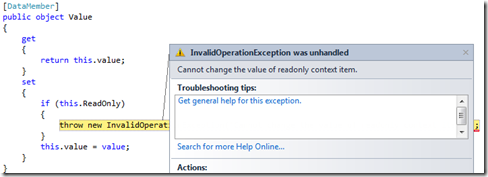# 一、问题重现

`   1: [DataContract(Namespace = "http://www.artech.com")]`
`   2: public class ContextItem`
`   3: {`
`   4:     private object value = null;`
`   5:     [DataMember]`
`   6:     public string Key { get; private set; }`
`   7:     [DataMember]`
`   8:     public object Value`
`   9:     {`
`  10:         get`
`  11:         {`
`  12:             return this.value;`
`  13:         }`
`  14:         set`
`  15:         {`
`  16:             if (this.ReadOnly)`
`  17:             {`
`  18:                 throw new InvalidOperationException("Cannot change the value of readonly context item.");`
`  19:             }`
`  20:             this.value = value;`
`  21:         }`
`  22:     }`
`  23:     [DataMember]`
`  24:     public bool ReadOnly { get; set; }`
`  25:     public ContextItem(string key, object value)`
`  26:     {`
`  27:         if (string.IsNullOrEmpty(key))`
`  28:         {`
`  29:             throw new ArgumentNullException("key");`
`  30:         }`
`  31:         this.Key = key;`
`  32:         this.Value = value;`
`  33:     }`
`  34: }`

`   1: public static T Deserialize<T>(string fileName)`
`   2: {`
`   3:     DataContractSerializer serializer = new DataContractSerializer(typeof(T));`
`   4:     using (XmlReader reader = new XmlTextReader(fileName))`
`   5:     {`
`   6:         return (T)serializer.ReadObject(reader);`
`   7:     }`
`   8: }`
`   9:  `
`  10: public static void Serialize<T>(T instance, string fileName)`
`  11: {`
`  12:     DataContractSerializer serializer = new DataContractSerializer(typeof(T));`
`  13:     using (XmlWriter writer = new XmlTextWriter(fileName, Encoding.UTF8))`
`  14:     {`
`  15:         serializer.WriteObject(writer, instance);`
`  16:     } `
`  17:     Process.Start(fileName);`
`  18: }`

`   1: static void Main(string[] args)`
`   2: {`
`   3:     var contextItem1 = new ContextItem("__userId", "Foo");`
`   4:     contextItem1.ReadOnly = true;`
`   5:     Serialize<ContextItem>(contextItem1, "context.xml");`
`   6:     var contextItem2 = Deserialize<ContextItem>("context.xml");           `
`   7: }`# 三、解决方案一：通过控制属性反序列化顺序

`   1: <ContextItem xmlns:i="http://www.w3.org/2001/XMLSchema-instance" xmlns="http://www.artech.com">`
`   2:     <Key>__userId</Key>`
`   3:     <ReadOnly>true</ReadOnly>`
`   4:     <Value xmlns:d2p1="http://www.w3.org/2001/XMLSchema" i:type="d2p1:string">Foo</Value>`
`   5: </ContextItem>`

`   1: [DataContract(Namespace = "http://www.artech.com")]`
`   2: public class ContextItem`
`   3: {`
`   4:     private object value = null;`
`   5:     private bool readOnly;`
`   6:     [DataMember]`
`   7:     public string Key { get; private set; }`
`   8:  `
`   9:     [DataMember(Order = 1)]`
`  10:     public object Value`
`  11:     {`
`  12:         get`
`  13:         {`
`  14:             return this.value;`
`  15:         }`
`  16:         set`
`  17:         {`
`  18:             if (this.readOnly)`
`  19:             {`
`  20:                 throw new InvalidOperationException("Cannot change the value of readonly context item.");`
`  21:             }`
`  22:             this.value = value;`
`  23:         }`
`  24:     }`
`  25:     [DataMember(Order =2)]`
`  26:     public bool ReadOnly`
`  27:     {`
`  28:         get`
`  29:         {`
`  30:             return readOnly;`
`  31:         }`
`  32:         set`
`  33:         {`
`  34:             readOnly = value;`
`  35:         }`
`  36:     }`
`  37:     //Others`
`  38: }`

# 四、解决方案二：将数据成员定义在字段上而不是属性上

`   1: [DataContract(Namespace = "http://www.artech.com")]`
`   2: public class ContextItem`
`   3: {`
`   4:     [DataMember]`
`   5:     public string Key { get; private set; }`
`   6:  `
`   7:     [DataMember(Name = "Value")]`
`   8:     private object value = null;`
`   9:     public object Value`
`  10:     {`
`  11:         get`
`  12:         {`
`  13:             return this.value;`
`  14:         }`
`  15:         set`
`  16:         {`
`  17:             if (this.ReadOnly)`
`  18:             {`
`  19:                 throw new InvalidOperationException("Cannot change the value of readonly context item.");`
`  20:             }`
`  21:             this.value = value;`
`  22:         }`
`  23:     }`
`  24:     [DataMember]`
`  25:     public bool ReadOnly { get; set; }     `
`  26:      //Others`
`  27:     }`
`  28: }`

# 总结